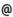## Babes-Bolyai University of Cluj-Napoca Faculty of Mathematics and Computer Science Study Cycle: Master SUBJECT

 Code Subject MM277 Special Topics in Fluid Mechanics
 Section Semester Hours: C+S+L Category Type Applied Mathematics 2 2+2+0 compulsory Mathematical Models in Mechanics and Astronomy - in English 1 2+2+0 compulsory
 Teaching Staff in Charge
 Prof. KOHR Mirela, Ph.D.,  mkohrmath.ubbcluj.ro
 Aims The aim of this course is to introduce and thoroughgoing study certain notions and fundamental results of fluid mechanics. The introduction in some special chapters of fluid mechanics with a special attention on the mathematical theory of viscous fluid flows at low Reynolds numbers. The presentation of some modern mathematical methods, such as the method of fundamental solutions and its applications in the theory of viscous incompressible fluid flows. The applications of the potential theory for Stokes, Stokes resolvent and Brinkman equations in the study of some viscous linearized flows in the presence of solid bodies, fluid interfaces, or in porous media. The presentation of some numerical methods that are useful in the tretment of the proposed problems, with a special attention on the boundary element method. The problems that will be treated have many applications in medicine, biology, chemical industry, geology, etc. The implication of the students in the research activity.
 Content 1. Fundamental notions and results of fluid mechanics. - Fluid, configuration, flow. - Velocity and acceleration fields of fluid flow (continuous medium) - Principle of the mass conservation. The continuity equation. - The Cauchy stress principle. The Cauchy fundamental theorem. - The principle of the rate of change of momentum. The Cauchy equation. 2. Constitutive equations of fluid mechanics. - The constitutive equation of ideal fluid. The Euler equations. - The constitutive equations of viscous Newtonian fluid. The Navier-Stokes equations. 3. Nondimensional analysis of the equations of viscous incompressible fluid flow - Special forms of the Navier-Stokes equations. - Boundary and initial conditions for the problem of viscous incompressible fluid flow. 4. Uniqueness results for the Stokes system. - Uniqueness result of the classical solution to the Stokes system in a bounded domain in Rⁿ (n=2,3). - Uniqueness result of the classical solution to the Stokes system in an unbounded domain in Rⁿ (n=2,3). 5. The method of fundamental solutions in fluid mechanics - The Green function, the pressure vector and the stress tensor for the Stokes flow due to a point force. The Oseen-Burgers tensor in Rⁿ (n=2, 3). - The direct boundary integral representation of the velocity field of a Strokes flow in a bounded or exterior domain in Rⁿ (n=2, 3). - Applications. 6. The potential theory for the Stokes equations. - The theory of compact operators. The Fredholm alternatives. The double-layer potential. The single-layer potential. Properties. - Applications of the potential theory for the Stokes system: Existence and uniqueness results to the boundary value problems for the Stokes system on bounded or exterior domains in Rⁿ (n≥2). Applications in the study of Stokes flows. - The completed double layer boundary integral equation method in the study of some Stokes flows. Existence and uniqueness results, as well as numerical results. 7. Boundary value problems for viscous incompressible flows at low Reynolds numbers in porous media or past porous bodies. - Existence and uniqueness results in Hölder or Sobolev spaces. - Applications and numerical results. 10. Numerical methods in the study of some problems concerning linearized viscous flows, with a special attention on the boundary element method.
 References 1. Kohr, M., Pop, I., Viscous Incompressible Flow for Low Reynolds Numbers, WIT Press (Wessex Institute of Technology Press)/Computational Mechanics Publications, Southampton (UK) - Boston, 2004 2. Kohr, M., Probleme Moderne în Mecanica Fluidelor Vâscoase, Presa Universitară Clujeană, Cluj-Napoca, 2 vols., 2000 3. Kohr, M., Studiul unor Mişcări Fluide Vâscoase Incompresibile prin Metode Integrale pe Frontieră, Presa Universitară Clujeană, Cluj-Napoca, 1997 4. Wendland, W.L., Hsiao, G.C., Boundary Integral Equations, Springer, Heidelberg, 2008 5. Dragoş, L., Mecanica Fluidelor, vol. I, Editura Academiei, Bucureşti, 1999 6. Truesdell, C., Rajagopal, K.R., An Introduction to the Mechanics of Fluids, Birkhäuser, Basel, 2000 7. Truesdell, C., A First Course in Rational Continuum Mechanics, Vol. 1, Academic Press, New York, 1991 8. Pozrikidis, C., Boundary Integral and Singularity Methods for Linearized Viscous Flow, Cambridge University Press, Cambridge, 1992 9. Pozrikidis, C., Introduction to Theoretical and Computational Fluid Dynamics, Oxford University Press, Oxford, 1997 10. Power, H., Wrobel, L.C., Boundary Integral Methods in Fluid Mechanics, WIT Press/Computational Mechanics Publications, Southampton (UK) – Boston, 1995 11. Varnhorn, W., The Stokes Equations, Akademie Verlag, Berlin, 1994.
 Assessment Exam (70%)+ student activity (30%).
 Links: Syllabus for all subjects Romanian version for this subject Rtf format for this subject